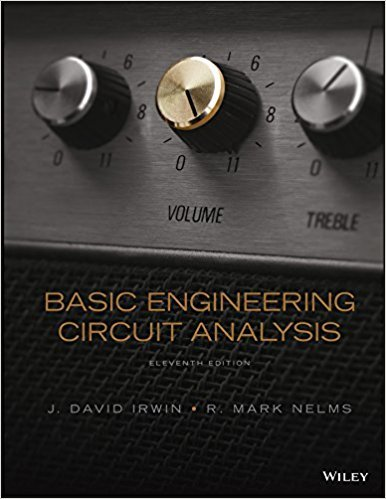×
Get Full Access to Basic Engineering Circuit Analysis - 11 Edition - Chapter 3 - Problem 3.65
Get Full Access to Basic Engineering Circuit Analysis - 11 Edition - Chapter 3 - Problem 3.65

×

# Find Io in the network in Fig. P3.65 using mesh analysis.ISBN: 9781118539293 159

## Solution for problem 3.65 Chapter 3

Basic Engineering Circuit Analysis | 11th Edition

• Textbook Solutions
• 2901 Step-by-step solutions solved by professors and subject experts
• Get 24/7 help from StudySoup virtual teaching assistantsBasic Engineering Circuit Analysis | 11th Edition

4 5 1 424 Reviews
19
1
Problem 3.65

Find Io in the network in Fig. P3.65 using mesh analysis. + + 6 V 6 k 24 V 6 k 4 k Io Figure P3.65

Step-by-Step Solution:
Step 1 of 3

Sample Notes EE 212 AC Steady State Power  Different types of AC power 1. Instantaneous Power [Watts] 2. Average Power (Active Power, Real Power) [Watts] 3. Reactive Power (Imaginary Power) [VAR] 4. Complex Power [VA] 5. Apparent Power [VA] 6. Power Factor  Instantaneous Power p(t) [Watts] p(t) = v(t) i(t); t = time 1. If current i(t) is entering the device then p(t) is positive and the device is absorbing power 2. If current i(t) is leaving the device then p(t) is negative and the device is supplying power

Step 2 of 3

Step 3 of 3

##### ISBN: 9781118539293

The full step-by-step solution to problem: 3.65 from chapter: 3 was answered by , our top Engineering and Tech solution expert on 11/23/17, 05:00AM. This textbook survival guide was created for the textbook: Basic Engineering Circuit Analysis, edition: 11. Basic Engineering Circuit Analysis was written by and is associated to the ISBN: 9781118539293. Since the solution to 3.65 from 3 chapter was answered, more than 353 students have viewed the full step-by-step answer. This full solution covers the following key subjects: analysis, fig, figure, Find, Mesh. This expansive textbook survival guide covers 15 chapters, and 1430 solutions. The answer to “Find Io in the network in Fig. P3.65 using mesh analysis. + + 6 V 6 k 24 V 6 k 4 k Io Figure P3.65” is broken down into a number of easy to follow steps, and 26 words.

Unlock Textbook Solution